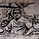# Indicators: Three included :: IFT on CCI, Z-Score and R-Squared

7198 görüntülenme
7198
*** Full description in the comment below ******

This chart has 3 indicators:
- Chande's R2
- Zscore
- Inverse Fisher Transform on CCI

Feel free to "make mine" (click on the SHARE button) and use these indicators in your charts.
```//
// @author LazyBear
// If you use this code in its orignal/modified form, do drop me a note.
//
study("Inverse Fisher Transform CCI [LazyBear]", shorttitle="IFTCCI_LB")
length = input(title="CCI Length", type=integer, defval=20)
src = close
cc=cci(src, length)

// Calculate IFT on CCI
lengthwma=input(9, title="Smoothing length")
calc_ifish(series, lengthwma) =>
v1=0.1*(series-50)
v2=wma(v1,lengthwma)
ifish=(exp(2*v2)-1)/(exp(2*v2)+1)
ifish

plot(calc_ifish(cc, lengthwma), color=teal, linewidth=1)
hline(0.5, color=red)
hline(-0.5, color=green)
hline(0)```Hi,

@LazyBear .....any chance that you can share R-Squared indicator?

"Make it Mine" option is unavailable under the share button for these sets of indicators.

thanks
Cevap GönderInverse Fisher Transform on CCI
-----------------------------------
Suggested by John Ehlers, IFT helps you to determine the exact oversold/overbought points in any oscillator-type indicators.

This chart has IFT on CCI.

Suggested method to use any IFT indicator is to buy when the indicator crosses over –0.5 or crosses over +0.5 if it has not previously crossed over –0.5 and to sell short when the indicators crosses under +0.5 or crosses under –0.5 if it has not previously crossed under +0.5.

Z-Score
-----------------------------------
The Z score is essentially the number of standard deviations the price is away from the mean price.

This means this is "related" to Bollinger Bands. If Z > 2, price is above the bands, if Z < -2, price is below the bands, assuming the bands are placed at 2 standard deviations. If your trading strategy uses bollinger bands and reacts to closing or crossing outside the bands, this indicator makes it very easy to see where you are in that range, just add 'levels' to the indicator at -2, 0, and 2.

R-Squared
-----------------------------------
R-Squared is a linear regression method that helps quantify the strength of market trends (i.e. “trendiness of prices). To determine if a trend is statistically significant for an n-periods linear regression line a 95% confidence level is required. The 95% confidence level varies based on the number of periods being evaluated. If the R-Squared value is less than its corresponding 95% confidence level for a given n-periods it is generally assumed that no statistically significant trend exists. The table below outlines the recommended number of R-Squared periods and their corresponding 95% confidence levels.

# of Periods / R2 Critical Value (95% Confidence)
5 / 77
10 / 40
14 / 27
20 / 20
25 / 16
30 / 13
50 / 8
60 / 6
120 / 3

One recommended trading method is to use R-Squared in conjunction with oscillators. Potential trading signals would be generated with respect to the oscillator’s overbought and oversold levels while R-Squared remains low (i.e. well below its 95% confidence level) indicating that prices are less “trendy”.

For additional information you may wish to refer to Tushar Chande’s and Stanley Kroll’s book entitled, “The New Technical Trader".
Cevap Gönder